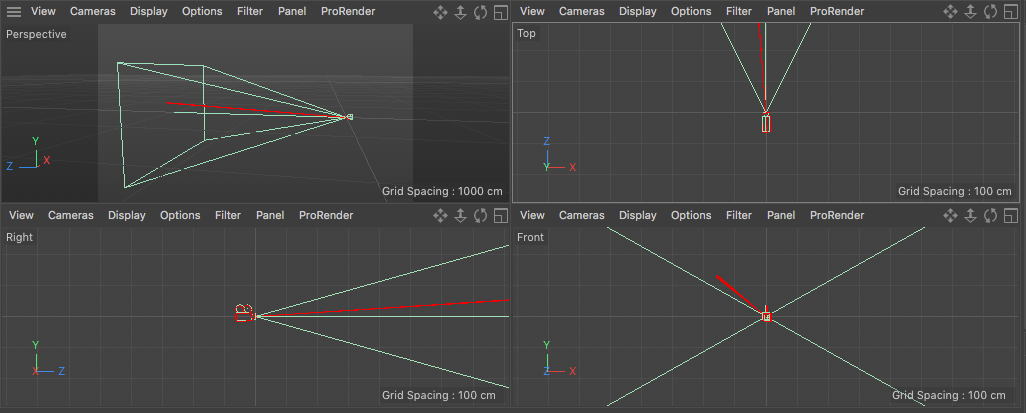# SOLVEDDirection vector

Hi folks,

I can't get this right. Sometime a go I posted a topic on CreateRay(). I got a (spherical?) example which was awesome, and I could play around with it to some degree. But I'm not grasping how I can expand on this.

Instead of a spherical lens, if I have two angles that I want to 'add' to my camera's rotation, how do I create a direction vector from the camera matrix with this? How would this look in math/coding terms with the following added to the camera rotation:

``````yaw = 4.5°
pitch = 3.7°
``````

I'm trying to create a direction vector that's based on two angles from my camera's centre. So 4.5° left/right and 3.7° up/down kind of thing.

WP.

Hi wickedP, thanks for reaching out us.

With regard to your request, the solution is pretty easy: using the GetRotationMatrixX() and GetRotationMatrixY() you get two transformation matrixes that in the end should be multiplied by your camera transformation matrix.In Python it looks like

``````    # get the cam
cam = doc.GetActiveObject()

#get the ray (it's just another camera with 0.2 aperture)
ray = cam.GetNext()

# create the yaw and pitch matrixes
pitchMatrix = c4d.utils.MatrixRotX(c4d.utils.DegToRad(3.7))
yawMatrix = c4d.utils.MatrixRotY(c4d.utils.DegToRad(4.5))

# combine them and set the ray tranformation matrix
ray.SetMg(pitchMatrix * yawMatrix * cam.GetMg())

c4d.EventAdd()
``````

Hi wickedP, thanks for reaching out us.

With regard to your request, the solution is pretty easy: using the GetRotationMatrixX() and GetRotationMatrixY() you get two transformation matrixes that in the end should be multiplied by your camera transformation matrix.In Python it looks like

``````    # get the cam
cam = doc.GetActiveObject()

#get the ray (it's just another camera with 0.2 aperture)
ray = cam.GetNext()

# create the yaw and pitch matrixes
pitchMatrix = c4d.utils.MatrixRotX(c4d.utils.DegToRad(3.7))
yawMatrix = c4d.utils.MatrixRotY(c4d.utils.DegToRad(4.5))

# combine them and set the ray tranformation matrix
ray.SetMg(pitchMatrix * yawMatrix * cam.GetMg())

c4d.EventAdd()
``````

Thanks Ricky,

but I'm not sure if that's what I'm after. That looks like normal object rotation, but I'm trying to make a direction vector. The example given in a previous post did something like this:

``````CreateRay(Ray* dst,Real x,Real y)
{
CameraMatrix = MyCam->GetMg();

Vector H = CameraMatrix.v1;
Vector V = CameraMatrix.v2;
Vector A = CameraMatrix.v3;
Vector direction_vector = H + V + A;

// how do I "add" 4.5° yaw to the direction_vector?
// similarly for pitch

dst->v = direction_vector;
dst->p = CameraMatrix.off;
}
``````

How do I "add" 4.5 degrees to the yaw with something like this (taking into account the camera's rotation as well)? I'd like to see the math so I can play around with that.

If it's easier, think of it like this - I'm trying to create the standard camera ray without any lens effects (trying to keep it simple). How is the standard camera ray done?

WP.